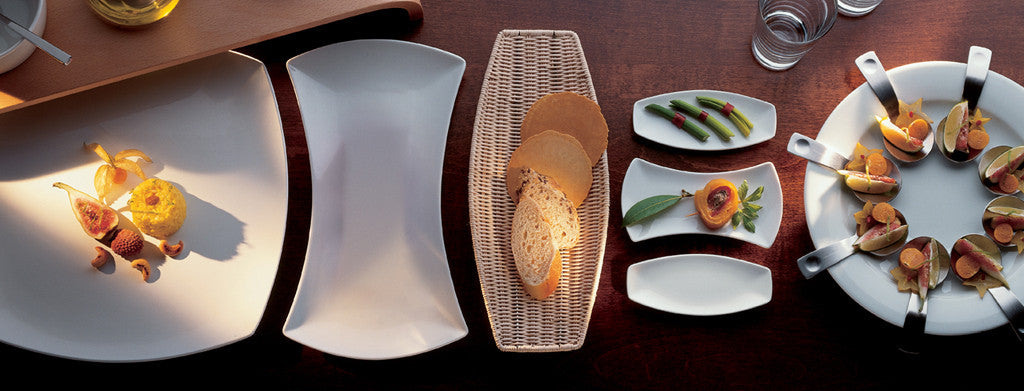Shopping Cart

### Refine

View all

#### Price# Crockery£4.69 each (ex VAT)£2.74 each (ex VAT)£3.54 each (ex VAT)£4.83 each (ex VAT)£5.93 each (ex VAT)£3.83 each (ex VAT)£3.23 each (ex VAT)£3.26 each (ex VAT)£5.12 each (ex VAT)£6.04 each (ex VAT)£4.27 each (ex VAT)£2.99 each (ex VAT)£2.07 each (ex VAT)£2.96 each (ex VAT)£5.54 each (ex VAT)£3.14 each (ex VAT)£7.14 each (ex VAT)£6.64 each (ex VAT)£5.70 each (ex VAT)£6.12 each (ex VAT)£4.06 each (ex VAT)£2.20 each (ex VAT)£3.26 each (ex VAT)£5.12 each (ex VAT)£3.76 each (ex VAT)£3.76 each (ex VAT)£16.49 each (ex VAT)£13.00 each (ex VAT)£7.72 each (ex VAT)£7.94 each (ex VAT)£6.27 each (ex VAT)£12.35 each (ex VAT)£9.60 each (ex VAT)£8.26 each (ex VAT)£15.03 each (ex VAT)£11.25 each (ex VAT)